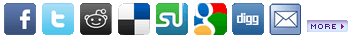Understanding How Microsoft Project Thinks Date: Thursday, August 21 @ 06:47:34 EDTTopic: PMConnection Articles There are a number of factors that dictate how Microsoft Project “thinks”. The two main factors are: The Scheduling Formula The Task Type The Scheduling Formula leverages three variables: Units - The percentage of time required by a resource or resources to complete the task Duration - The number of working days required to complete the task Work - The effort required to complete the task Many people say that the scheduling formula is: Units x Duration = Work When you initially assign a Resource to a Task, this is the formula that is leveraged. Let’s demonstrate: We will create Task 1 that is 5 days in Duration We will now assign a Resource to this task As soon as we assign this Resource to this task, Work changes to 40 hours. Here is the how that number was calculated: Units x Duration = Work100% x (5*8) = 40Or100% x 40 = 40 hours But using simple math, we can re-write our equation to solve for a different variable. Our original formula solves for Work:Units x Duration = Work But we could re-write the formula to solve for Units:Work / Duration = Units Or we could re-write the formula to solve for Duration:Work / Units = Duration So we have shown that The Scheduling Formula can actually be written three different ways: Units x Duration = WorkWork / Duration = UnitsWork / Units = Duration Now let’s prove that Microsoft Project also thinks this way. In order to demonstrate this, we must introduce The Task Type or the Type field: By default, Type is set to Fixed Units. But there are actually three different Task Type variables: Fixed Duration Fixed Units Fixed Work Now to test Microsoft Project Using our original scenario, let’s force Microsoft Project to solve for Duration. We will leave Type set to Fixed Units and change Work to 80 hrs Here is how Duration was calculated: Work / Units = Duration80 / 100% = 80Or 80 / 100% = (80 / 8)Or80 / 100% = 10 days Returning to our original scenario, let’s force Microsoft Project to solve for Work. Leave Type set to Fixed Units and enter 10 days Duration Here is how Work was calculated: Units x Duration = Work100% * 10 days = 80Or 100% * 10 days = (10 * 8)Or100% * 10 days = 80 hours Work Returning to our original scenario, let’s force Microsoft Project to solve for Units Change Type to Fixed Duration and enter 20 hours Work Here is how Units was calculated: Work / Duration = Units20 / 5 days = 50%Or20 / 5 days = 20 / (8 * 5)Or20 / 5 days = 20 / 40Or20 / 5 days = 0.5 To Review: There are three different Task Types: Fixed Units Fixed Work Fixed Duration There are three different variables of the Scheduling Formula: Units Work Duration Depending upon how the Task Type is set in combination with which Scheduling Formula variable is altered dictates which variable Microsoft Project solves for. The Task Type and Scheduling Formula Cheat Sheet will help you control how Microsoft Project “thinks”: See The Microsoft Project Task Type and Scheduling Formula Cheat Sheet here. See this article in Slide format here. See this article in Video format here. Purchase “The Cheat Sheet” Mouse Pad here.